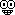SB Physics Help Room Sucks!
Would you like to react to this message? Create an account in a few clicks or log in to continue.

# Post Quiz 22.1 Help Here##Post Quiz 22.1 Help Here

hey guys feel free to post any solutions/help on 22.1 here!

k8
Guest

##Question 1

An object is placed 75.1 cm from the surface of a plane mirror. What is the distance between the image and the mirror surface (see sheet 3) ? Indicate with a positive (negative) sign whether the image is real and in front of the mirror (virtual and behind the mirror).

Just convert the distance the object is from the surface of the plane mirror into meters and that is your answer. See the reasoning for it on sheet 3. Answer is (-) because it is not a real image, it is virtual, there is no light behind the mirror. In this example, the answer would be -.751

Kathleen

Posts : 42
Join date : 2008-12-18

##Question 2

The radius of curvature of the surface of a convex spherical mirror is 62.04 cm. What is the magnitude of the focal length (see sheet 5,9) ? Be sure to include the correct sign of the focal length.

F = R/2, where F is the focal length, R is the radius in meters.
Convert your radius to meters (62.04cm -> .6204 m) and solve for f
F= .6204/2 = .3102
Answer is negative though I'm not certain as to why just yet.

Kathleen

Posts : 42
Join date : 2008-12-18

##Question 3

An object is placed 54.65 cm from the surface of a spherical mirror with a (-38.62 cm) focal length. How far from the mirror surface is the image (see sheet 11,12) ? Indicate with a positive (negative) sign whether the image is inverted and real (upright and virtual)

NOTE: for this first part keep everything in centimeters..you can convert at the end
You're going to use the equation from sheet 12 part C where (1/i) = (1/f)-(1/o)
You are given f = -38.62 cm, and o=54.65 cm
(1/i) = (1/-38.62)-(1/54.65)
(1/i) = -.04419
(1/.04419) = i
i = 22.629 cm
Now you can convert, so this is .22629 m

Kathleen

Posts : 42
Join date : 2008-12-18

##Question 4

An object is placed 9.932 cm from the surface of a concave spherical mirror with a 34.47 cm radius of curvature. What is the magnification (see sheet 5,7,8,9) ? Make sure your sign is correct. Remember that negative magnification means the image is inverted; if the absolute value of the magnification is less than one, then the image is smaller than the object.

There are a couple of steps for this:
First, find the focal length of the mirror

F = R/2
Where R=34.47 cm
F = 34.47/2 = 17.235

Next, find i
(1/i) = (1/f) - (1/o)
(1/i) = (1/17.235) - (1/9.932)
(1/i) = -.04266
(1/-.04266) = -23.439 cm

Now convert that to m and you get -23.439cm -> -.23439 m
Convert the object distance to meters also, 9.932 cm -> .09932 m

Finally, solve for magnification
m = -(i/o)
m= -(-.23439/.09932)
m = 2.3599 m

This is the answer and it is positive

Kathleen

Posts : 42
Join date : 2008-12-18

##Thanks!

Thank you for your assistance kathleen!!!

k8
Guest

##THANKS

Thanks Kathleen!!! Your da best!Money23
Guest

##Re: Post Quiz 22.1 Help Here